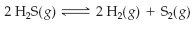×
Get Full Access to Introductory Chemistry - 5 Edition - Chapter 15 - Problem 68p
Get Full Access to Introductory Chemistry - 5 Edition - Chapter 15 - Problem 68p

×

# Get answer: Consider the effect of a volume change on this reaction at equilibriumISBN: 9780321910295 34

## Solution for problem 68P Chapter 15

Introductory Chemistry | 5th Edition

• Textbook Solutions
• 2901 Step-by-step solutions solved by professors and subject experts
• Get 24/7 help from StudySoup virtual teaching assistantsIntroductory Chemistry | 5th Edition

4 5 1 261 Reviews
25
3
Problem 68P

Problem 68P

Consider the effect of a volume change on this reaction at equilibrium.Predict the effect (shift right, shift left, or no effect) of these changes.

(a) increasing the reaction volume

(b) decreasing the reaction volume

Step-by-Step Solution:

Solution 68P:

Here, we are going describe the the effect of a volume change on this reaction at equilibrium.

Step 1:

Le Châtelier’s principle states that when a chemical system at equilibrium is disturbed by changing the concentration, pressure or temperature, then the system shifts in a direction that minimizes the disturbance.

Step 2 of 4

Step 3 of 4

##### ISBN: 9780321910295

The full step-by-step solution to problem: 68P from chapter: 15 was answered by , our top Chemistry solution expert on 05/06/17, 06:45PM. Since the solution to 68P from 15 chapter was answered, more than 313 students have viewed the full step-by-step answer. The answer to “Consider the effect of a volume change on this reaction at equilibrium. Predict the effect (shift right, shift left, or no effect) of these changes.(a) increasing the reaction volume(b) decreasing the reaction volume” is broken down into a number of easy to follow steps, and 33 words. This textbook survival guide was created for the textbook: Introductory Chemistry, edition: 5. Introductory Chemistry was written by and is associated to the ISBN: 9780321910295. This full solution covers the following key subjects: Volume, effect, reaction, shift, left. This expansive textbook survival guide covers 19 chapters, and 2046 solutions.

Unlock Textbook Solution